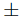# JEE(MAIN) Chemistry Mock Test - 8

## 30 Questions MCQ Test JEE Main Mock Test Series 2021 & Previous Year Papers | JEE(MAIN) Chemistry Mock Test - 8

Description
This mock test of JEE(MAIN) Chemistry Mock Test - 8 for JEE helps you for every JEE entrance exam. This contains 30 Multiple Choice Questions for JEE JEE(MAIN) Chemistry Mock Test - 8 (mcq) to study with solutions a complete question bank. The solved questions answers in this JEE(MAIN) Chemistry Mock Test - 8 quiz give you a good mix of easy questions and tough questions. JEE students definitely take this JEE(MAIN) Chemistry Mock Test - 8 exercise for a better result in the exam. You can find other JEE(MAIN) Chemistry Mock Test - 8 extra questions, long questions & short questions for JEE on EduRev as well by searching above.
QUESTION: 1

Solution:
QUESTION: 2

Solution:
QUESTION: 3

### The rate, at which a substance reacts at constant temperature, depends upon its

Solution:
QUESTION: 4
The pH of blood is around
Solution:
QUESTION: 5
The indicator that is obtained by coupling the diazonium salt of sulphanilic acid with N,N-dimethylaniline is
Solution:
QUESTION: 6
What will be the weight of deposited silver on passing 965 coulombs of electricity in solution of AgNO₃?
Solution:
QUESTION: 7
Which of the following is an extensive property?
Solution:
QUESTION: 8
For the reaction A→B, the rate law expression is rate = k[A]. Which of the following statements is incorrect?
Solution:
QUESTION: 9
AgCl is soluble in NH₄OH solution. The solubility is due to formation of
Solution:
QUESTION: 10
The name of the element with atomic number 100 was adopted in honour of
Solution: Fermium (symbol Fm and atomic number 100) was named for the American physicist Enrico Fermi. It is a synthetic, radioactive, metallic transuranic element of the actinide series.
QUESTION: 11
Which one of the following is the strongest acid?
Solution:
QUESTION: 12
Corrosion can be prevented by
Solution:
QUESTION: 13
Which one of the following can be considered as weak electrolyte ?
Solution:
QUESTION: 14

Which of the following is not true in case of reaction with heated copper at 300oC?

Solution:
QUESTION: 15
A mixture of a weak acid (say acetic acid )and its salt with a strong base (say sodium acetate )is a buffer solution.Which other pair of substances from the following may have a similar property ?
Solution:
QUESTION: 16

Which of the following is a Lewis acid?

Solution:

"Lewis Acid is something that accepts a pair of electrons."

"A Lewis Base is something that donates a pair of electrons."

So Cl- would be a Lewis base.

NH3 is a Lewis Base.

BF3 is NOT a Lewis Base because it has an empty p orbital, this is a Lewis Acid.

C2H5OH is ethanol and that is a Lewis Base because the Oxygen has two lone pairs of electrons that it can donate.

QUESTION: 17
Orlon is a polymer of
Solution:
QUESTION: 18
Ethyl bromide treatements with alcoholic KOH gives
Solution:
QUESTION: 19

Which does not contain conjugate system?

Solution:

In dienes, when the double bonds connected to each other by a single bond, this arrangement is known as conjugated.

QUESTION: 20

The crystal system of a compound with unit cell dimensions a=0.387, b=0.387 and c=0.0504 nm and α = β = 90and γ = 120is

Solution:
QUESTION: 21
Crystals of covalent compounds always have
Solution:
QUESTION: 22
Which one of the following solutions of sulphuric acid will exactly neutralise 25 ml of 0.2 M sodium hydroxide solution ?
Solution:
QUESTION: 23
The molarity of pure water is
Solution:
QUESTION: 24
When an alkyne,RC ≡ CH, is treated with cuprous ion in an ammoniacal medium,one of the product is
Solution:
QUESTION: 25

What is the wavelength of the electron in the nth Bohr's orbit?

Solution:

λ = 2 π r

Where, r = radius of the Bohr's first orbit for nth orbit =
∴ λ = (2 x n x 3.146 x 0.529)Å

QUESTION: 26

The quantum number of most energetic electron in Ne atom when it is in first excited state is__

Solution:

The electronic configuration of Ne atom is 1s2 2s2 2p6. In its first excitation state the electron from 2p level will unpair and shift to 3s where quantum number will be 3,0,0,1/2

QUESTION: 27

For a system in equilibrium ,∆G = 0 under conditions of constant

Solution:
QUESTION: 28

The frequancy factor in the collision theory is__

Solution: Frequency factor in collision theory is directly proportional to √T because,as temperature increases, molecules move faster; as molecules move faster, they are more likely to collide and therefore affect the collision frequency
QUESTION: 29

Among the following metal carbonyls, the C --- O bond order is lowest in :

Solution:

Mn+ = 3d54s1 in presence of CO effective configuration = 3d64s0.
Three lone pair for back bonding with vacant orbital of C in CO. So, it has maximum bond order..

Fe0 = 3d64s2 in presence of CO effective configuration = 3d8 four lone pair for back bonding with CO.

Cr0 = 3d54s1
Effective configuration = 3d6. Three lone pair for back bonding with CO.

V− = 3d44s2 effective configuration = 3d6three lone pair for back bonding with CO.

Maximum back bonding is present in Fe(CO)5 therefore CO bond order is lowest here.

QUESTION: 30

If 0.32 g of an organic compound containing sulphur produces 0.233g of BaSO₄. Then the percentage of sulphur in it is

Solution: